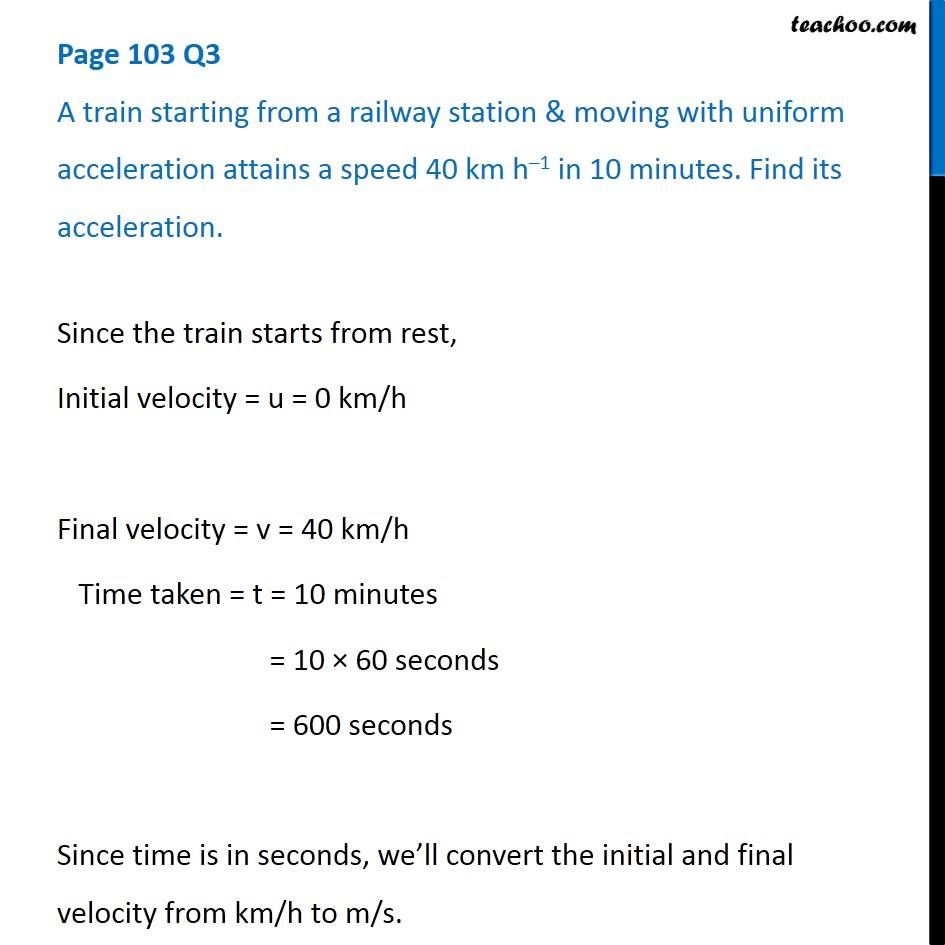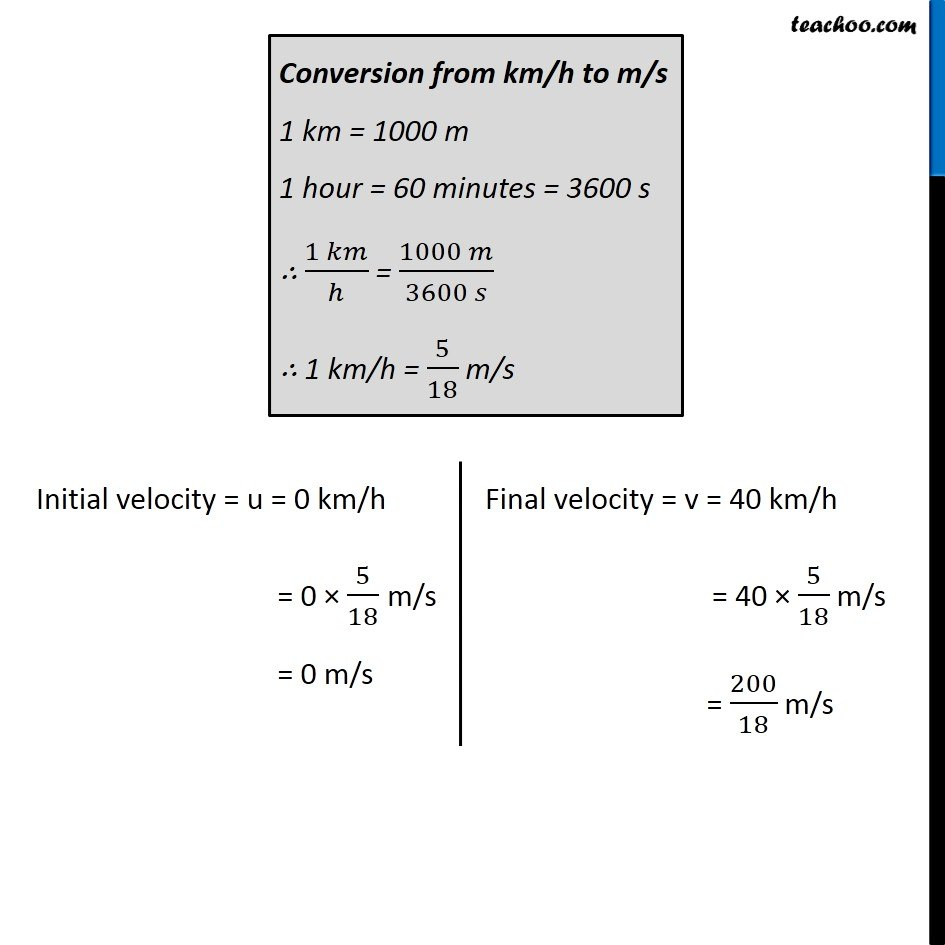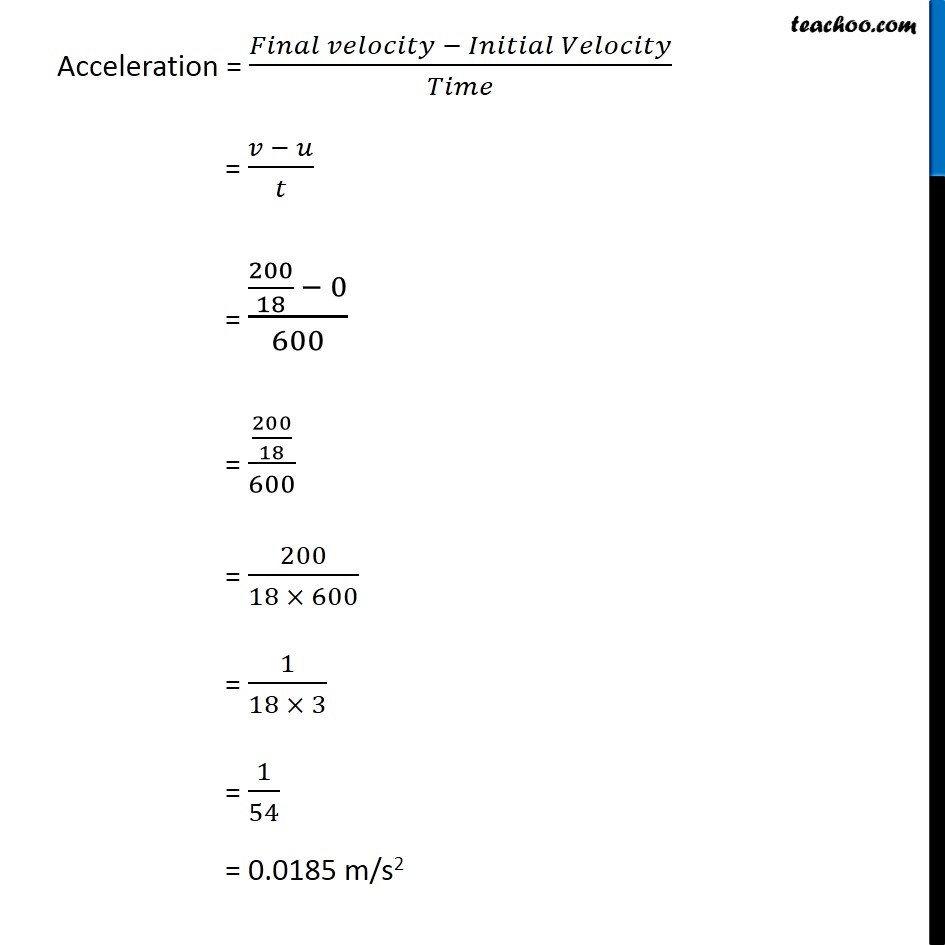Questions from Inside the chapter

Class 9
Chapter 8 Class 9 - MotionLearn in your speed, with individual attention - Teachoo Maths 1-on-1 Class

### Transcript

Page 103 Q3 A train starting from a railway station & moving with uniform acceleration attains a speed 40 km h–1 in 10 minutes. Find its acceleration. Since the train starts from rest, Initial velocity = u = 0 km/h Final velocity = v = 40 km/h Time taken = t = 10 minutes = 10 × 60 seconds = 600 seconds Since time is in seconds, we’ll convert the initial and final velocity from km/h to m/s. Conversion from km/h to m/s 1 km = 1000 m 1 hour = 60 minutes = 3600 s ∴ (1 𝑘𝑚)/ℎ = (1000 𝑚)/(3600 𝑠) ∴ 1 km/h = 5/18 m/s Initial velocity = u = 0 km/h = 0 × 5/18 m/s = 0 m/s Final velocity = v = 40 km/h = 40 × 5/18 m/s = 200/18 m/s Acceleration = (𝐹𝑖𝑛𝑎𝑙 𝑣𝑒𝑙𝑜𝑐𝑖𝑡𝑦 − 𝐼𝑛𝑖𝑡𝑖𝑎𝑙 𝑉𝑒𝑙𝑜𝑐𝑖𝑡𝑦)/𝑇𝑖𝑚𝑒 = (𝑣 − 𝑢)/𝑡 = (200/18 − 0)/600 = (200/18)/600 = 200/(18 × 600) = 1/(18 × 3) = 1/54 = 0.0185 m/s2